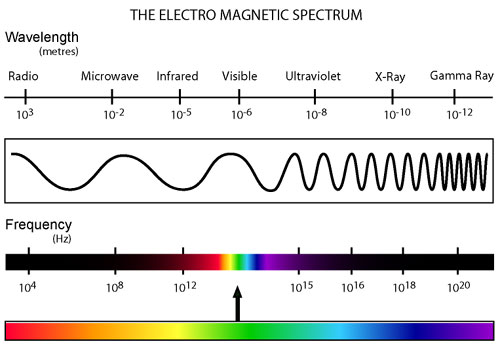Skip to main content

# 1. The Electromagnetic Spectrum

The electromagnetic spectrum is the entire range of frequencies (or wavelengths) of light. Light, which Maxwell’s equations showed to be electromagnetic waves (radiation) propagated from a source of electric and magnetic fields, can be treated as both a wave and particle (see Basics of Quantum Theory); thus, while we think of light as particles, it helps to view the spectrum of light in terms of waves. The wavelength of light, or the distance from one high peak to the next in the light wave, becomes shorter as the energy of the photon becomes higher; the frequency, which is the number of wave cycles per unit time, becomes higher because there are more oscillations in a given amount of time. The closer the peaks are together, the more energetic the photon is.

Key points about the electromagnetic spectrum:

• Longer wavelength (and therefore lower frequency) corresponds to less energy, while shorter wavelength and higher frequency mean more energy.
• The spectrum consists of all kinds of light, classified by ranges of wavelengths. Radio waves, microwaves, visible light, etc. are all different types of light that are set between two specific wavelengths. For example, visible light is between 390 and 750 nanometers (a nanometer is a billionth of a meter).
• As can be seen by the illustration below, the visible light (light that we can see) only makes up a small portion of light that exists; all around us is light that our eyes cannot interpret! For example, only when you don a pair of infrared goggles can you see the infrared wavelengths emanating from the heat of our bodies. Similarly, only when you turn on a radio do you detect radio waves.Source: <effieboo.wordpress.com/2010/0...etic-spectrum/>

• Was this article helpful?